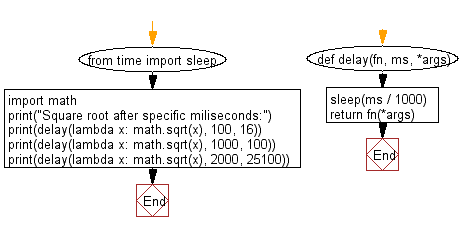﻿ Python Exercise: Invoke a given function after specific milliseconds - w3resource# Python Exercise: Invoke a given function after specific milliseconds

## Python Functions: Exercise - 21 with Solution

Write a Python program that invoke a given function after specific milliseconds.

Sample Solution:-

Python Code:

``````from time import sleep
import math
def delay(fn, ms, *args):
sleep(ms / 1000)
return fn(*args)
print("Square root after specific miliseconds:")
print(delay(lambda x: math.sqrt(x), 100, 16))
print(delay(lambda x: math.sqrt(x), 1000, 100))
print(delay(lambda x: math.sqrt(x), 2000, 25100))
```
```

Sample Output:

```Square root after specific miliseconds:
4.0
10.0
158.42979517754858
```

Flowchart:## Visualize Python code execution:

The following tool visualize what the computer is doing step-by-step as it executes the said program:

Python Code Editor:

Have another way to solve this solution? Contribute your code (and comments) through Disqus.

What is the difficulty level of this exercise?

Test your Programming skills with w3resource's quiz.

﻿

## Python: Tips of the Day

Decapitalizes the first letter of a string:

Example:

```def tips_decapitalize(s, upper_rest=False):
return s[:1].lower() + (s[1:].upper() if upper_rest else s[1:])
print(tips_decapitalize('PythonTips'))
print(tips_decapitalize('PythonTips', True))
```

Output:

```pythonTips
pYTHONTIPS
```